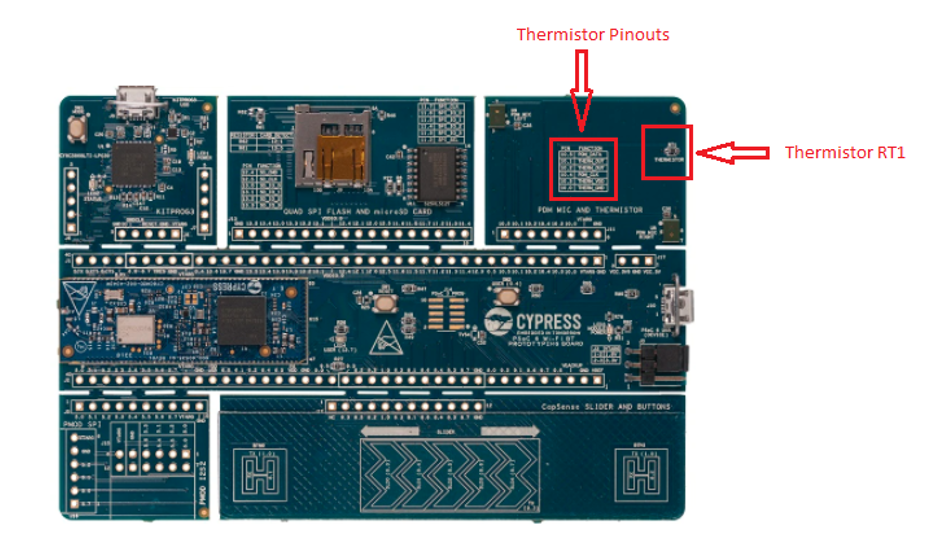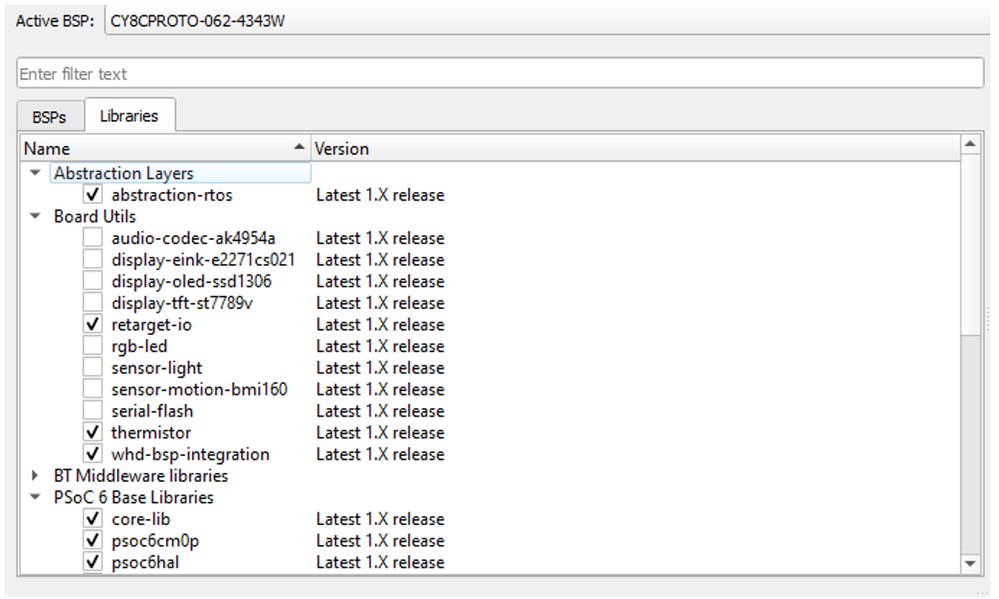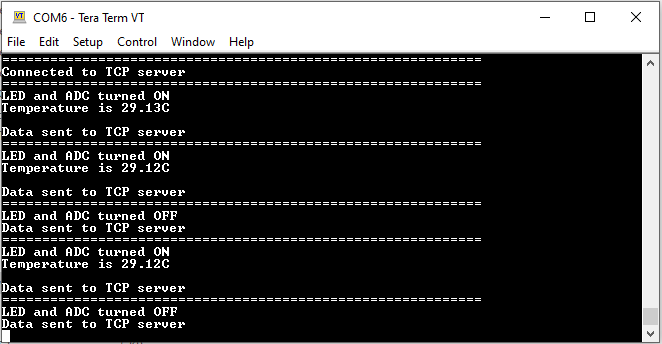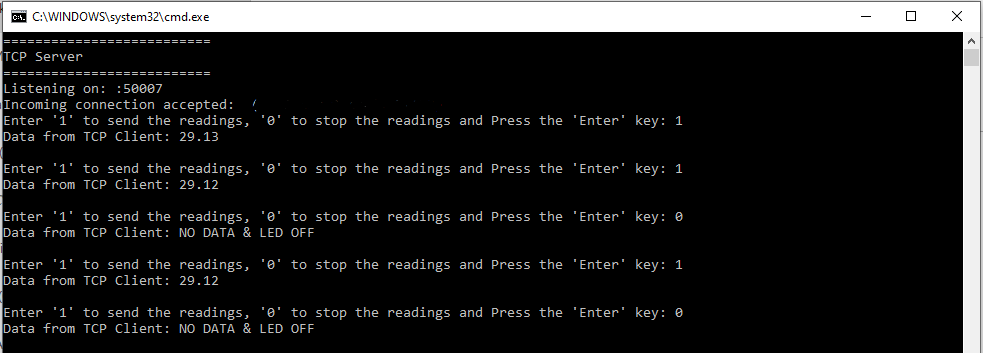cancel
Showing results for
Did you mean:

# ModusToolbox BlogModerator

## Integrating Thermistor with the TCP client using Thermistor libraries on ModusToolbox

In this project, we will be integrating the onboard thermistor with the AnyCloud TCP Client project in the Modus Toolbox. We will be using thermistor libraries and their respective APIs to work with the onboard thermistor.

Hardware setup

PSoC 6 Wi-Fi BT Prototyping Kit (CY8CPROTO-062-4343W)The thermistor is connected to PSoC 6 pin 10.1 and 10.2, as shown on the board.

Software setup

1. We will start by creating the AnyCloud TCP Client project in the MODUS Toolbox. We can create the project by clicking on New Application in Quick Panel. Select the BSP as CY8CPROTO-062-4343W Kit. Select the AnyCloud TCP Client, you can rename the project if needed. Click on Create.
2. Now as we have created the TCP Client project, we need to add the libraries which will be required for this project. In this project, we will be requiring a thermistor library and to do that, click on Library Manager under Tools in the Quick Panel and select thermistor (under Board Utils) as shown.After successfully adding the library, we need to include the library in the project i.e. #include “mtb_thermistor_ntc_gpio.h”.

Description

The project contains three major source files -

• main.c
• tcp_client.c
• tcp_client.h

In tcp_client.h we are adding few marcros, which are:

#define THERM_GND   P10_0

#define THERM_VDD   P10_3

#define THERM_OUT   P10_2

NOTE: For CY8CPROTO-62-4343W Kit, we need to swap the GND and VCC pin macros as there is a slight error in the hardware. Hence, the macros for the PROTO kit would look like:

#define THERM_GND   P10_3

#define THERM_VDD   P10_0

#define THERM_OUT   P10_2

In tcp_client.c we are adding the following code:

The thermistor used in this kit is NCP18XH103F03RB. It follows negative temperature coefficient (NTC) characteristics i.e. the resistance decreases with an increase in temperature.

The software flow is as follows:

1. After adding the include files, we need to create few structures for the thermistor as:

mtb_thermistor_ntc_gpio_t mtb_thermistor_obj;

/* Refer thermistor datasheet for the values */

mtb_thermistor_ntc_gpio_cfg_t therm_gpio_cfg=

{

.b_const = (float)(3380), // Refers to beta constant

.r_infinity = (float)(0.1192855),  //Refers to resistance at infinity

/**Resistance of the thermistor is 10K at 25 degrees C (from datasheet). Therefore R0 = 10000 Ohm, and T0 = 298.15 Kelvin, which gives  R_INFINITY = R0 e^(-B_CONSTANT / T0) = 0.1192855 **/

.r_ref = (float)(10000)           // Refers to reference resistance

};

All the above structures will be used in the thermistor APIs for temperature calculations. Ideally, we initialize them as global.

2. Next, we will initialize the thermistor using the pre-defined APIs as:

result = mtb_thermistor_ntc_gpio_init (&mtb_thermistor_obj, &adc_obj, THERM_GND,

THERM_VDD, THERM_OUT, &therm_gpio_cfg);

We need to first initialize ADC, and for that, we are using the HAL API of the ADC i.e. cyhal_adc_init () as shown. It takes three arguments, reference to ADC structure, ADC pin (THERM_OUT), and clock. Three GPIO pins of the PSoC are being used here, which are:

• THERM_OUT

As shown on the board, this is an analog pin 10.1 or 10.2, where the thermistor is connected

• THERM_VDD

This is a GPIO pin which will be pulled high acting as VDD for thermistor.

• THERM_GND

This is a GPIO pin which will be pulled low acting as ground for thermistor.

All these GPIO initialization are done in terms of MACROS in tcp_client.h file.

After ADC initialization, we initialize thermistor GPIO i.e. mtb_thermistor_ntc_gpio_init (). It takes six arguments, reference to thermistor structure, reference to ADC structure, VDD pin, GND pin, Output/ADC pin, and reference to thermistor configuration structure.

3. We are done with the initialization and we shall use the temperature API, which accepts the readings got from ADC and converts them into temperature (degree centigrade).

value = mtb_thermistor_ntc_gpio_get_temp(&mtb_thermistor_obj);

The above API takes one argument as reference to thermistor object and the API returns the floating-point value which is the actual measured temperature in degree centigrade.

4. After getting the values, we simply pass the values into the tcp_client_recv_handler() function, which will simply print the values on UART (i.e. TCP Client) w.r.t. the command received from the TCP Server.

5. If in TCP server, the command sent is “1” then the TCP Client will print the value of thermistor on UART and will acknowledge the TCP Server by sending the same value to it and simultaneously will turn the LED and ADC On. Similarly, if the command is “0”, TCP Client will not send the readings and will turn the LED and ADC OFF as shown in the below figures.Figure: TCP Client (UART)Figure: TCP Server (Python)